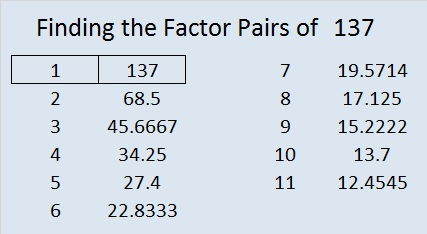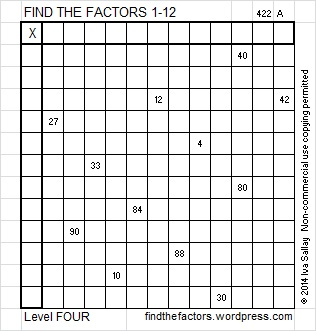# 137 and Level 4

• 137 is a prime number.
• Prime factorization: 137 is prime.
• The exponent of prime number 137 is 1. Adding 1 to that exponent we get (1 + 1) = 2. Therefore 137 has exactly 2 factors.
• Factors of 137: 1, 137
• Factor pairs: 137 = 1 x 137
• 137 has no square factors that allow its square root to be simplified. √137 ≈ 11.7046999How do we know that 137 is a prime number? If 137 were not a prime number, then it would be divisible by at least one prime number less than or equal to √137 ≈ 11.7. Since 137 cannot be divided evenly by 2, 3, 5, 7, or 11, we know that 137 is a prime number.

137 is never a clue in the FIND THE FACTORS puzzles.Excel file with puzzles and the previous week’s factor solutions: 12 Factors 2014-06-02